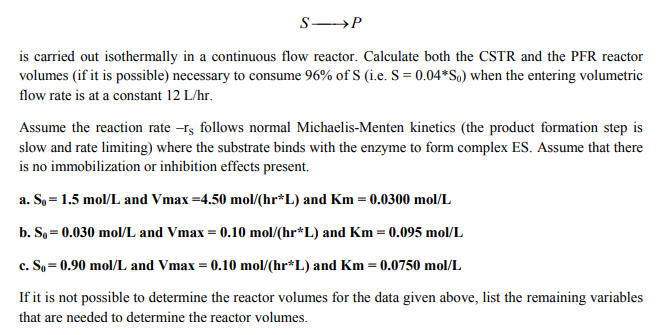# is carried out isothermally in a continuous flow reactor. Calculate both the CSTR and the PFR reactorvolumes (if it is possible) necessary to consurme 96% of S (ie. S = 0.04 *S) when the entering volumetricflow rate is at a constant 12 L/hr.Assume the reaction rate -s follows normal Michaelis-Menten kinetics (the product formation step isslow and rate limiting) where the substrate binds with the enzyme to form complex ES. Assume that thereis no immobilization or inhibition effects present.a. So-1.5 mol/L and Vmax-4.50 mol/(hr L) and Km- 0.0300 mol/Lb. S,-0.030 mol/L and Vmax = 0.10 mol/(hr*L) and Km = 0.095 mol/Lc. So= 0.90 mol/L and Vmax 0.10 mol/(hr*L) and Km = 0.0750 mol/Llfitismotpossibletodetermine thereactor vol esforthedata given above, l tthe remai ingvariablesthat are needed to determine the reactor volumes.

Question
109 viewshelp_outlineImage Transcriptioncloseis carried out isothermally in a continuous flow reactor. Calculate both the CSTR and the PFR reactor volumes (if it is possible) necessary to consurme 96% of S (ie. S = 0.04 *S) when the entering volumetric flow rate is at a constant 12 L/hr. Assume the reaction rate -s follows normal Michaelis-Menten kinetics (the product formation step is slow and rate limiting) where the substrate binds with the enzyme to form complex ES. Assume that there is no immobilization or inhibition effects present. a. So-1.5 mol/L and Vmax-4.50 mol/(hr L) and Km- 0.0300 mol/L b. S,-0.030 mol/L and Vmax = 0.10 mol/(hr*L) and Km = 0.095 mol/L c. So= 0.90 mol/L and Vmax 0.10 mol/(hr*L) and Km = 0.0750 mol/L lfitismotpossibletodetermine thereactor vol esforthedata given above, l tthe remai ingvariables that are needed to determine the reactor volumes. fullscreen
check_circle

Step 1

The reaction taking place is,

S→P

According to the Michaelis-Menten equation, the reaction rate for this reaction is defined as written below.

Step 2

For CSTR, the volume of the reactor is calculated by the formula derived below:

Here,

VCSTR = volume of the reactor

= volumetric flowrate

[S]0 = Initial substrate concentration

Step 3

For PFR, the volume of the reactor is calculated by the formula derived below:

Here,

VPFR = volume of the reactor

V̇...

### Want to see the full answer?

See Solution

#### Want to see this answer and more?

Solutions are written by subject experts who are available 24/7. Questions are typically answered within 1 hour.*

See Solution
*Response times may vary by subject and question.
Tagged in

### Chemical Engineering